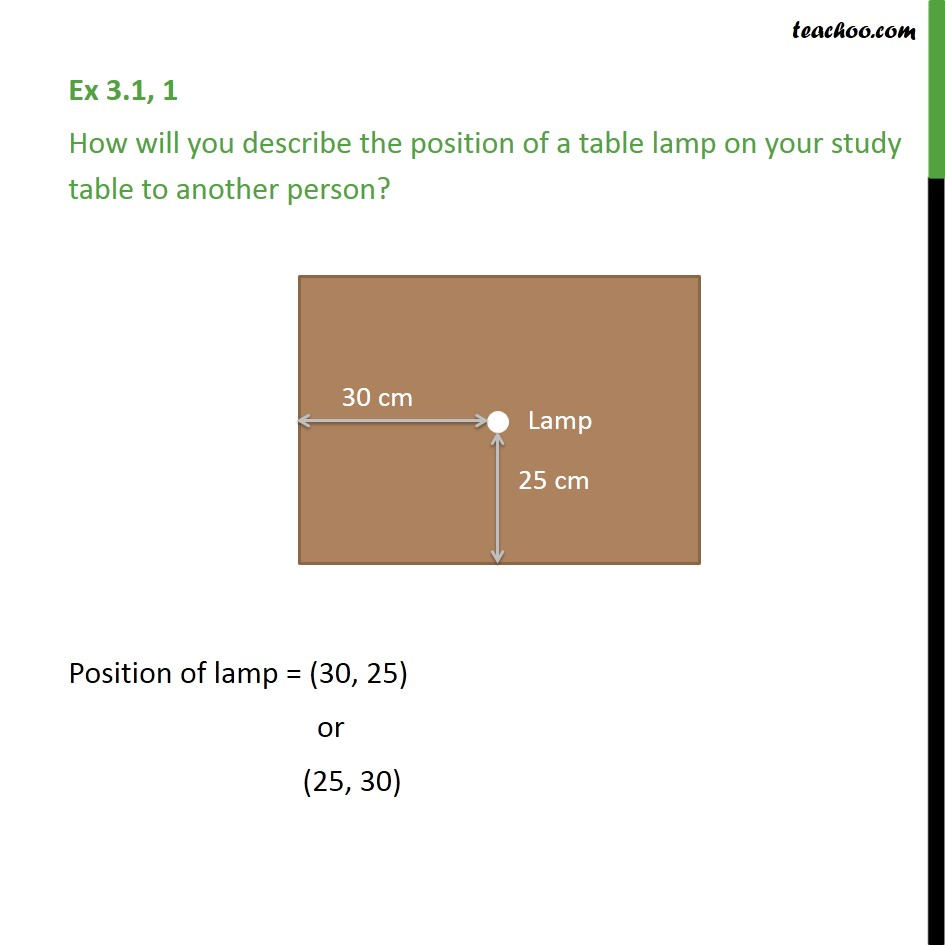Learn all Concepts of Coordinate Geometry Class 9 (with VIDEOS). Check - Coordinate Geometry Class 91. Chapter 3 Class 9 Coordinate Geometry
2. Concept wise
3. Activities

Transcript

Ex 3.1, 1 How will you describe the position of a table lamp on your study table to another person? To describe the position of lamp, we find its distance from bottom edge and left edge We notice tthat distance from left edge is 30 cm and from bottom edge is 25 cm Thus, Position of lamp = (30, 25) or (25, 30)

Activities